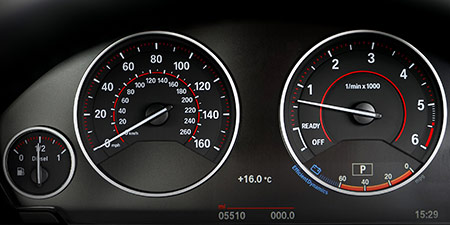# Meters per second (m/s) to Yards per hour (yd/h)

Thema Units of speed ﹣ Converter Meters per second to Yards per hour

Here you can convert the Speed unit Meters per second into the unit Yards per hour and vice versa you can convert Yards per hour into Meters per second. By clicking the "Swap units" icon, you will always obtain the desired conversion in the calculation result, i.e., m/s to yd/h or yd/h to m/s. With the following calculator you can also calculate any other Speed unit.

## Info about "Meters per second"

Meters per second is a basic unit for speed in the International System of Units (SI) and other metric systems. This is because both meter and second are the international base units for distance and time. Definition of "meters per second": An object moving at 1 "m/s" per second will cover a distance of one meter.

In our daily work, speed is commonly measured in km/h. The following rule is an easy way to remember when converting m/s into km/h: &qquot; multiply by four and minus 10 %". For example, standard walking speeds in meters per second are about 1 m/s for a walker, 10.44 m/s is the world record for running 100 meters, and the speed of sound is 343 meters per second.

As mentioned above, both meter and second are the international base units for distance and time. All other speed units in the metric system are derived based on meters per second (e.g. km/h, mm/s).

## Info about "Yards per hour"

The length unit "Yard" is commonly used in the Anglo-American countries. For example, speeds are represented by yard per unit of time.

The unit is defined as follows: An object moving at 1 "Yard per hour" per hour will cover a distance of one yard. Therefore, 1 yard is equivalent to 0.9144 meters (3 feet).

## Basis for conversion Meters per second (m/s) to Yards per hour (yd/h) and vice versa

The abbreviation for the "Speed unit Meters per second" is m/s. The abbreviation for the "Speed unit Yards per hour" is yd/h.

## Formula for the conversion of Meters per second (m/s) to Yards per hour (yd/h) and vice versa

The calculation from Meters per second to Yards per hour shall be made using the following conversion formula:

Conversion formula Meters per second to Yards per hour

Determine the number of Yards per hour from Meters per second

Meters per second × 3937.0078740158

## Formula for the conversion of Yards per hour (yd/h) to Meters per second (m/s)

The calculation from Yards per hour to Meters per second shall be made using the following conversion formula:

Conversion formula Yards per hour to Meters per second

Determine the number of Meters per second from Yards per hour

Yards per hour × 0.000254

## Overview table : How many Meters per second are in Yards per hour ?

Meters per second m/s ⇒ Yards per hour yd/h
0.01 m/s  are  39.37007 yd/h
0.02 m/s  are  78.74015 yd/h
0.03 m/s  are  118.11023 yd/h
0.04 m/s  are  157.48031 yd/h
0.05 m/s  are  196.85039 yd/h
0.06 m/s  are  236.22047 yd/h
0.07 m/s  are  275.59055 yd/h
0.08 m/s  are  314.96063 yd/h
0.09 m/s  are  354.33070 yd/h
0.10 m/s  are  393.70078 yd/h
0.20 m/s  are  787.40157 yd/h
0.30 m/s  are  1 181.10236 yd/h
0.40 m/s  are  1 574.80315 yd/h
0.50 m/s  are  1 968.50393 yd/h
0.60 m/s  are  2 362.20472 yd/h
0.70 m/s  are  2 755.90551 yd/h
0.80 m/s  are  3 149.60629 yd/h
0.90 m/s  are  3 543.30708 yd/h
1 m/s  corresponds to  3 937.00787 yd/h
2 m/s  are  7 874.01574 yd/h
3 m/s  are  11 811.02362 yd/h
4 m/s  are  15 748.03149 yd/h
5 m/s  are  19 685.03937 yd/h
6 m/s  are  23 622.04724 yd/h
7 m/s  are  27 559.05511 yd/h
8 m/s  are  31 496.06299 yd/h
9 m/s  are  35 433.07086 yd/h
10 m/s  are  39 370.07874 yd/h
20 m/s  are  78 740.15748 yd/h
30 m/s  are  118 110.23622 yd/h
40 m/s  are  157 480.31496 yd/h
50 m/s  are  196 850.39370 yd/h
60 m/s  are  236 220.47244 yd/h
70 m/s  are  275 590.55118 yd/h
80 m/s  are  314 960.62992 yd/h
90 m/s  are  354 330.70866 yd/h
100 m/s  are  393 700.78740 yd/h
200 m/s  are  787 401.57480 yd/h
300 m/s  are  1 181 102.36220 yd/h
400 m/s  are  1 574 803.14960 yd/h
500 m/s  are  1 968 503.93700 yd/h
600 m/s  are  2 362 204.72440 yd/h
700 m/s  are  2 755 905.51181 yd/h
800 m/s  are  3 149 606.29921 yd/h
900 m/s  are  3 543 307.08661 yd/h
1 000 m/s  are  3 937 007.87401 yd/h

## Overview table : How many Yards per hour are in Meters per second ?

Yards per hour yd/h ⇒ Meters per second m/s
0.04 yd/h  are  0.00001 m/s
0.05 yd/h  are  0.00001 m/s
0.06 yd/h  are  0.00001 m/s
0.07 yd/h  are  0.00001 m/s
0.08 yd/h  are  0.00002 m/s
0.09 yd/h  are  0.00002 m/s
0.10 yd/h  are  0.00002 m/s
0.20 yd/h  are  0.00005 m/s
0.30 yd/h  are  0.00007 m/s
0.40 yd/h  are  0.00010 m/s
0.50 yd/h  are  0.00012 m/s
0.60 yd/h  are  0.00015 m/s
0.70 yd/h  are  0.00017 m/s
0.80 yd/h  are  0.00020 m/s
0.90 yd/h  are  0.00022 m/s
1 yd/h  corresponds to  0.00025 m/s
2 yd/h  are  0.00050 m/s
3 yd/h  are  0.00076 m/s
4 yd/h  are  0.00101 m/s
5 yd/h  are  0.00127 m/s
6 yd/h  are  0.00152 m/s
7 yd/h  are  0.00177 m/s
8 yd/h  are  0.00203 m/s
9 yd/h  are  0.00228 m/s
10 yd/h  are  0.00254 m/s
20 yd/h  are  0.00508 m/s
30 yd/h  are  0.00762 m/s
40 yd/h  are  0.01016 m/s
50 yd/h  are  0.01270 m/s
60 yd/h  are  0.01524 m/s
70 yd/h  are  0.01778 m/s
80 yd/h  are  0.02032 m/s
90 yd/h  are  0.02286 m/s
100 yd/h  are  0.02540 m/s
200 yd/h  are  0.05080 m/s
300 yd/h  are  0.07620 m/s
400 yd/h  are  0.10160 m/s
500 yd/h  are  0.12700 m/s
600 yd/h  are  0.15240 m/s
700 yd/h  are  0.17780 m/s
800 yd/h  are  0.20320 m/s
900 yd/h  are  0.22860 m/s
1 000 yd/h  are  0.25400 m/s

## Source information

As source for the information in the "Units of speed" category, we have used in particular:

## Last update on May 3, 2022

The pages of the "Units of speed" category were last editorially reviewed by Stefan Banse on May 3, 2022. They all correspond to the current status.

### Previous changes on October 9, 2021

• November 9, 2020: Publication of the Speed converter
• Editorial revision of all texts in this category